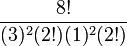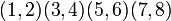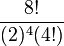# Difference between revisions of "Symmetric group:S8"

View a complete list of particular groups (this is a very huge list!)[SHOW MORE]

## Definition

This group is a finite group defined as the symmetric group on a set of size$8$. The set is typically taken to be$\{ 1,2,3,4,5,6,7,8 \}$.

In particular, it is a symmetric group on finite set as well as a symmetric group of prime power degree.

## Arithmetic functions

Function Value Similar groups Explanation
order (number of elements, equivalently, cardinality or size of underlying set) 40320 groups with same order The order is$8! = 8 \cdot 7 \cdot 6 \cdot 5 \cdot 4 \cdot 3 \cdot 2 \cdot 1$
exponent of a group 840 groups with same order and exponent of a group | groups with same exponent of a group The exponent is the least common multiple of$1,2,3,4,5,6,7,8$
Frattini length 1 groups with same order and Frattini length | groups with same Frattini length

## Elements

Further information: element structure of symmetric group:S8

### Upto conjugacy

Partition Verbal description of cycle type Representative element Size of conjugacy class Formula for size Even or odd? If even, splits? If splits, real in alternating group? Element orders
1 + 1 + 1 + 1 + 1 + 1 + 1 + 1 all points fixed$()$ -- the identity element 1$\frac{8!}{(1)^8(8!)}$ even;no 1
2 + 1 + 1 + 1 + 1 + 1 + 1 transposition, six fixed points$(1,2)$ 28$\frac{8!}{(2)(1)^6(6!)}$, also$\binom{8}{2}$ odd 2
3 + 1 + 1 + 1 + 1 + 1 one 3-cycle, five fixed points$(1,2,3)$ 112$\frac{8!}{(3)(1)^5(5!)}$ even;no 3
4 + 1 + 1 + 1 + 1 one 4-cycle, four fixed points$(1,2,3,4)$ 420$\frac{8!}{(4)(1)^4(4!)}$ odd 4
2 + 2 + 1 + 1 + 1 + 1 two transpositions, four fixed points$(1,2)(3,4)$ 210$\frac{8!}{(2)^2(2!)(1)^4(4!)}$ even;no 2
5 + 1 + 1 + 1 one 5-cycle, three fixed points$(1,2,3,4,5)$ 1344$\frac{8!}{(5)(1)^3(3!)}$ even;no 5
3 + 2 + 1 + 1 + 1 one 3-cycle, one transposition, three fixed points$(1,2,3)(4,5)$ 1120$\frac{8!}{(3)(2)(1)^3(3!)}$ odd 6
6 + 1 + 1 one 6-cycle, two fixed points$(1,2,3,4,5,6)$ 3360$\frac{8!}{(6)(1)^2(2!}$ odd 6
4 + 2 + 1 + 1 one 4-cycle, one 2-cycle, two fixed points$(1,2,3,4)(5,6)$ 2520$\frac{8!}{(4)(2)(1)^2(2!)}$ even;no 4
2 + 2 + 2 + 1 + 1 three 2-cycles, two fixed points$(1,2)(3,4)(5,6)$ 420$\frac{8!}{(2)^3(3!)(1)^2(2!)}$ odd 2
3 + 3 + 1 + 1 two 3-cycles, two fixed points$(1,2,3)(4,5,6)$ 1120$\frac{8!}{(3)^2(2!)(1)^2(2!)}$ even;no 3
7 + 1 one 7-cycle, one fixed point$(1,2,3,4,5,6,7)$ 5760$\frac{8!}{(7)(1)}$ even;yes;no 7
3 + 2 + 2 + 1 one 3-cycle, two transpositions, one fixed point$(1,2,3)(4,5)(6,7)$ 1680$\frac{8!}{(3)(2)^2(2!)(1)}$ even;no 6
4 + 3 + 1 one 4-cycle, one 3-cycle, one fixed point$(1,2,3,4)(5,6,7)$ 3360$\frac{8!}{(4)(3)(1)}$ odd 12
5 + 2 + 1 one 5-cycle, one 2-cycle, one fixed point$(1,2,3,4,5)(6,7)$ 4032$\frac{8!}{(5)(2)(1)}$ odd 10
2 + 2 + 2 + 2 four 2-cycles$(1,2)(3,4)(5,6)(7,8)$ 105$\frac{8!}{(2)^4(4!)}$ even;no 2
4 + 2 + 2 one 4-cycle, two 2-cycles$(1,2,3,4)(5,6)(7,8)$ 1260$\frac{8!}{(4)(2)^2(2!)}$ odd 4
3 + 3 + 2 two 3-cycles, one 2-cycle$(1,2,3)(4,5,6)(7,8)$ 1120$\frac{8!}{(3)^2(2!)(2)}$ odd 6
6 + 2 one 6-cycle, one 2-cycle$(1,2,3,4,5,6)(7,8)$ 3360$\frac{8!}{(6)(2)}$ even;no 6
5 + 3 one 5-cycle, one 3-cycle$(1,2,3,4,5)(6,7,8)$ 2688$\frac{8!}{(5)(3)}$ even;yes;no 15
4 + 4 two 4-cycles$(1,2,3,4)(5,6,7,8)$ 1260$\frac{8!}{(4)^2(2!)}$ even;no 4
8 one 8-cycle$(1,2,3,4,5,6,7,8)$ 5040$\frac{8!}{8}$ odd 8

## GAP implementation

Description Functions used
SymmetricGroup(8) SymmetricGroup